# 17 Using Trigonometry to Find Side Lengths

Click play on the following audio player to listen along as you read this section.

Our goal in this section is to take the information given to us regarding a triangle and find the missing side. Take a look at the triangle below and note the identified angle.

Given the identified angle we can then name each of the three sides. If we know one of those sides we could find the other two.

This is where we use trigonometry. Let’s say the side we know is the opposite side which has a value of 7. And then let’s say the side we wanted to find out was the hypotenuse.

In order to solve for the hypotenuse we first decide which of the three trigonometry formulas works for us. Keep in mind that the two sides we are dealing with are the opposite (which we have) and the hypotenuse (which we need to find).

 SOH CAH TOA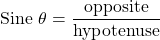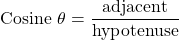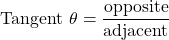From the three formulas we can see that the sine formula will work as it deals with the opposite and the hypotenuse. Now we need to rework the formula to solve for the hypotenuse.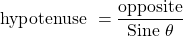The next step is to plug in the numbers and get an answer.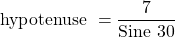So far so good except what do we do with the “sine 30”. That’s not a number we can work with.

Well sine 30 is the number we get from using trigonometry. What this represents is the relationship between the opposite and the hypotenuse with the identified angle being 30 degrees.

What we need to do now is plug that into our calculator to get the number that represents sine 30. What you should find on your calculator in a button labelled “SIN”.

This stands for sine. Now hit that button and enter 30. What do you get? Check out below to see if you get the same thing.

So the sine of 30 degrees is 0.5. Here it is mathematically.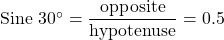Mathematically what this is saying is if the identified angle is 30 degrees then the relationship between the opposite and the hypotenuse is 0.5. Essentially this means that the opposite side is 0.5 times the length of the hypotenuse whenever the identified angle is 30 degrees. We can now solve for the hypotenuse.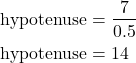Let’s take that same triangle we used up above and instead of trying to find the hypotenuse we’ll find the adjacent side.In this case we know what the opposite side is and we are looking to find the adjacent side. Once again let’s look at the three trigonometry functions and see which one works for us.

 SOH CAH TOAIn this case we can use the tangent function. Take that formula and solve for the adjacent side.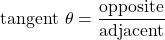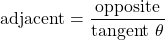Sometimes you might see tangent written as just “TAN” in the formula. No worries though. It means the same thing as tangent.

Now plug the numbers in.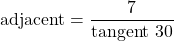Go to your calculator and put in the tangent of 30.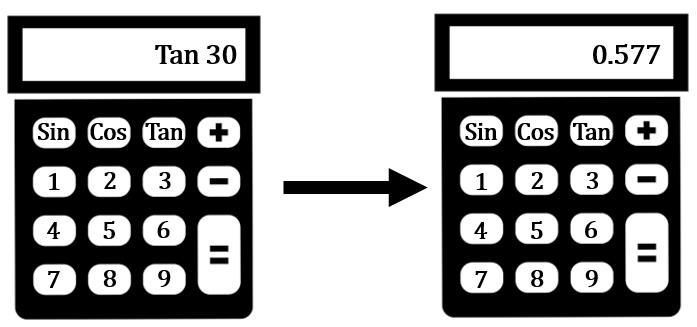Here we are getting the relationship between the opposite and the adjacent side given that the identified angle is 30 degrees. What the number is saying is  the opposite side is 0.577 times the length of the adjacent side. Now we can go ahead and calculate the adjacent side.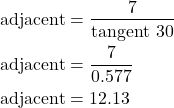There you have it. We knew the identified angle was 30 degrees and we had one of the sides. From that we were able to use trigonometry to find the other two sides.

But wait! We used the sine function and the tangent function to find the missing sides but we didn’t need to use the cosine function. Maybe we should take a look at the cosine function and use the same identified angle of 30 degrees and work with that.

First of all let’s take a look at the cosine function.

 SOH CAH TOAThe cosine function requires that we have either the adjacent or the hypotenuse. I know that at this point we know both of those but for the purpose of math let’s use the adjacent to find the hypotenuse. First let’s rearrange the formula to find the hypotenuse.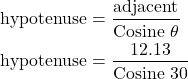From this point we need to go back to our calculator to find  the cosine of 30 degrees. Remember that this number represents the relationship between the adjacent side and the hypotenuse.

Take that number and plug it in to the formula and see what you get.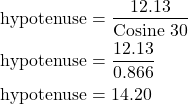We are off by just a little as we had previously calculated that the hypotenuse was 14. This slight difference is due to rounding during our calculations. In the end of the day we used all three trigonometry formulas (or trigonometric functions) to solve for the sides of the triangle.

At this point we’ll do a couple more examples before letting you tackle a few on your own.

Example

Find the length of side B given that side A is 14 and the identified angle is 60 degrees.

Step 1: Figure out which of the three trigonometry formulas you will be using.

In this case side A is the opposite side and side B is the adjacent side. We also know that the identified angle is 60 degrees. We will use tangent as we know the opposite side and need to find the adjacent side.Step 2: Rework the formula to solve for the adjacent side.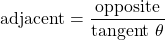Step 3: Plug in the numbers and solve.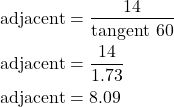Example

Solve for side C if side A is 17 and the identified angle is 23 degrees.

Step 1: Figure out which of the three trigonometry formulas you’ll be using.

In this case side B is the opposite side and side A is the adjacent side. We are looking to find side C which is the hypotenuse. We know the identified angle is 23 degrees and we know that the adjacent side is 17. We will end up using cosine so solve this question.Step 2: Rework the formula to solve for the hypotenuse.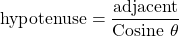Step 3: Plug in the numbers and solve.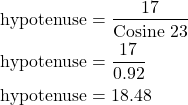Now try a couple questions for yourself. Make sure to check the video answers once you are done.

# Practice Questions